# Study Guide

## MathematicsSample Questions

### Competency 0001Understand real numbers and mathematical problem solving.

1. Two numbers have a greatest common factor of 60 and a least common multiple of 27,000. If one of these two numbers is 1,500, then what is the other number?

1. 450
2. 540
3. 900
4. 1,080
Correct Response: D. Use prime factorization to express the GCF as 2 squared times 3 times 5, the LCM as 2 cubed times 3 cubed times 5 cubed, and 1,500 as 2 squared times 3 times 5 cubed. The LCM contains the greatest power of any prime factor found in either number. Powers in the LCM that are not also common to those in 1,500 must be unique to the unknown number. Those powers are 2 cubed times 3 cubed. The GCF indicates that one factor of 5 is found in the unknown number. Multiply 2 cubed times 3 cubed times 5 to get 1,080.

### Competency 0002Understand complex numbers, vectors, and matrices.

2. A market share analysis shows that of the people who buy body wash, 40% currently buy Brand A, 35% currently buy Brand B, and 25% currently buy other brands. A satisfaction survey shows the following:

• 80% of those who buy Brand A say they will continue to buy it, 15% say they will buy Brand B, and 5% will buy other brands.
• Of those who currently buy Brand B, 10% will change to Brand A, 75% will continue to buy Brand B, and 15% will try another brand.
• Of the users of other brands, 20% will buy Brand A and 10% will buy Brand B.

If the current market share is represented by the 1 by 3 matrix 0.4, 0.35, and 0.25, which of the following matrix multiplications calculates the new market share for each brand?

1.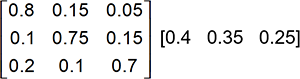The left matrix is 3 by 3. The elements by row are 0.8, 0.15, 0.05 0.1, 0.75, 0.15 0.2, 0.1, and 0.7. The right matrix is 1 by 3. The elements are 0.4, 0.35, and 0.25.
2.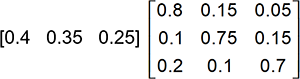The left matrix is 1 by 3. The elements are 0.4, 0.35, and 0.25.The right matrix is 3 by 3. The elements by row are 0.8, 0.15, 0.05, 0.1, 0.75, 0.15, 0.2, 0.1, and 0.7.
3.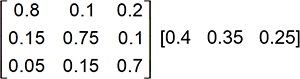The left matrix is 3 by 3. The elements by row are 0.8, 0.1, 0.2 0.15, 0.75, 0.1 0.05, 0.15, and 0.7. The right matrix is 1 by 3. The elements are 0.4, 0.35, and 0.25.
4.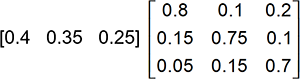The left matrix is 1 by 3. The elements are 0.4, 0.35, and 0.25. The right matrix is 3 by 3. The elements by row are 0.8, 0.1, 0.2 0.15, 0.75, 0.1 0.05, 0.15, and 0.7.
Correct Response: B. The matrix 1 by 3 matrix 0.4, 0.35, and 0.25 describes the current market share of the three brand categories by column, where Brand A has a market share of 0.4, or 40%. Based on the results of the survey, Brand A expects to retain 80% of the customers that make up its current market share, gain 10% of the market share held by Brand B, and gain 20% of the market share held by other brands. This new market share may be expressed as 0.4 times 0.8 plus 0.35 times 0.1 plus 0.25 times 0.2. Similarly, the expressions 0.4 times 0.15 plus 0.35 times 0.75 plus 0.25 times 0.1 and 0.4 times 0.05 plus 0.35 times 0.15 plus 0.25 times 0.7 describe the expected new market shares for Brand B and for other brands, respectively. These expressions are described by the matrix multiplication in this response.

### Competency 0003Understand relations and functions.

3. Which of the following sets represents the range of the function f of x equals the square root of open paren 9 minus x squared close paren?

1. the set of real numbers greater than or equal to 0
2. the set of real numbers less than or equal to 3
3. the set of real numbers greater than or equal to 0 and less than or equal to 3
4. the set of real numbers greater than or equal to negative 3 and less than or equal to 3
Correct Response: C. The range of a function represents all possible values that a function may return from any of the values allowed by its domain. The function f of x equals the square root of open paren 9 minus x squared close paren may take on any value of x that does not result in a negative radicand, which occurs when 9 minus x squared is greater than or equal to 0, which transforms to x squared is less than or equal to 9, which has the solution x is less than or equal to 3 and x is greater than or equal to negative 3. If the domain of negative 3 is less than or equal to x is less than or equal to 3, then the range of the function f of x is 0 is less than or equal to the function f of x is less than or equal to 3.

### Competency 0004Understand linear, quadratic, and higher-order polynomial functions.

4.Use the diagram below to answer the question that follows.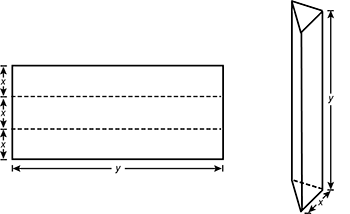A rectangular sheet is shown on the left. Dashed lines parallel to the bottom edge divide the rectangle into 3 segments that are long and narrow. The height of each segment is labeled x. The width of the rectangle is labeled y.
An equilateral triangular prism is shown to the right. An edge along the bottom face is labeled x and the height is labeled y.

A rectangular piece of sheet metal with a perimeter of 20 feet is folded on two parallel and equally spaced lines to form a prism whose ends are equilateral triangles. The prism will be a form for a concrete pillar. Which of the following functions expresses the volume of the form in terms of x, the distance in feet between the parallel fold lines?

1. V of x equals x squared times one fourth times the quantity 10 minus 3 x
2. V of x equals x squared times the square root of 3 times one fourth times the quantity 10 minus 3 x
3. V of x equals x squared times one fourth times the quantity 20 minus 6 x
4. V of x equals x squared times the square root of 3 times one fourth times the quantity 20 minus 6 x
Correct Response: B. The volume of a prism is equal to the area of the base times the length of the prism. The area of the base for this prism is the area of an equilateral triangle with side lengths of x. If an altitude is drawn from one vertex of the triangle, the properties of 30 degrees - 60 degrees - 90 degrees triangles can be applied to find the height of the equilateral triangle, which is x times the square root of 3 times one half. The area of the base of the prism is then one half times x times x times the square root of 3 times one half equals x squared times the square root of 3 times one fourth. The perimeter of the rectangular piece of sheet metal is equal to 6 x plus 2 y, so 6 x plus 2 y equals 20, and solving this equation for y gives to the length of prism in terms of x. The volume of the prism is equal to the area of the base of the prism times the length of the prism, which is one fourth times x squared times the square root of 3 times the quantity 10 minus 3 x.

### Competency 0005Understand measurement principles and procedures.

5. A company packages their oats in two sizes of similar cylindrical containers. The linear scale factor between the containers is 3:2. If the smaller container holds 38 ounces of oats, approximately how many ounces of oats are in the larger container?

1. 128 ounces
2. 114 ounces
3. 85 ounces
4. 57 ounces
Correct Response: A. The linear scale factor 3:2 means that the lengths of the radius and height of the larger container are three halves times greater than the corresponding lengths of the smaller container. If the smaller container has a volume of V equals pi times r squared times h equals 38 ounces, then the larger container has a volume of V equals pi times open paren three halves r close paren squared times open paren three halves times h close paren equals open paren three halves close paren cubed times 38 is approximately 128 ounces.

### Competency 0006Understand Euclidean geometry in two and three dimensions.

6. Use the diagram below to answer the question that follows.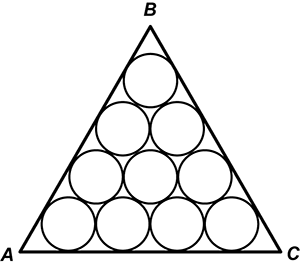The circles are congruent and packed in a triangular pattern such that the centers of circles in a row are aligned with the tangent points between the circles in neighboring rows.
The vertices of the triangle are labeled ABC, where AC is positioned along the bottom of the triangle with a horizontal alignment. The bottom row has 4 circles, and each successive row has 1 less circle.

Ten circles are drawn in equilateral triangle ABC so that the circles are tangent to each other and nine of them are tangent to the sides of the triangle. If each circle has a diameter of 4, how long is side AC?

1. 8 plus 4 times the square root of 3
2. 12 plus 2 times the square root of 3
3. 12 plus 4 times the square root of 3
4. 16 plus 2 times the square root of 3
Correct Response: C. The lines tangent to the circle centered at O, shown below,are perpendicular to its radii, and line segment A O is the hypotenuse of two right triangles.Angle X A Y is formed outside of the circle such that segments A X and A Y are tangent to the circle. Segment A O is shown. The radii, O X and O Y, are congruent. The radius of the circle is 2. Segment A Y is equal to two times the square root of three.
The two triangles are congruent by the Hypotenuse-Leg theorem, and it then follows that the triangles are 30 degrees - 60 degrees - 90 degrees triangles because angle O A X is congruent to angle O A Y and the measure of angle O A X plus the measure of angle O A Y is equal to 60 degrees. The leg opposite 60 degrees is square root of 3 times larger than the leg opposite 30 degrees, so A Y equals 2 times the square root of three, and the length of AC is equal to two of these segments and 3 circle diameters: two times the square root of three plus three times four plus 2 times the square root of three or twelve plus 4 times the square root of three.

### Competency 0007Understand coordinate and transformational geometry.

7. Which of the following transformations applied to the graph of y equals x cubed results in the graph of y equals negative open paren x minus 2 close paren cubed minus 1?

1. a horizontal shift 2 units to the left, followed by a vertical shift 1 unit downward, and then a reflection over the x-axis
2. a horizontal shift 2 units to the right, followed by a reflection over the x-axis, and then a vertical shift 1 unit downward
3. a horizontal shift 2 units to the left, followed by a vertical shift 1 unit upward, and then a reflection over the y-axis
4. a horizontal shift 2 units to the right, followed by a reflection over the y-axis, and then a vertical shift 1 unit downward
Correct Response: B. Translating the function y equals x cubed using a horizontal shift two units to the right creates the function y equals open paren x minus 2 close paren cubed. A reflection of this function over the x-axis changes the sign of all outputs, so the new function is y equals negative open paren x minus 2 close paren cubed. A vertical shift 1 unit downward decreases every output by 1, so the resulting function is y equals negative open paren x minus 2 close paren cubed minus 1.

### Competency 0008Understand trigonometric functions.

8. Use the diagram below to answer the question that follows.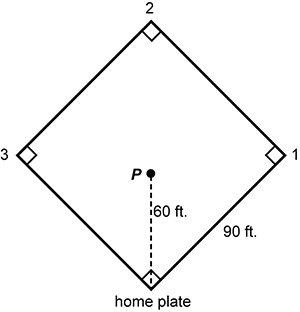The diamond in the shape of a square is oriented such that its diagonals have vertical and horizontal alignments. The bottom vertex is labeled home plate, and moving counterclockwise, the other vertices are labeled 1, 2 and 3. Right angles are shown at each vertex, and a dash line extends along the diagonal of the square from home plate to point P. That line is labeled 60 feet, and an edge of the square is labeled 90 feet.

A baseball diamond is a square with 90-foot sides and the bases at the corners. The pitcher's mound is on a diagonal 60 feet from home plate. Approximately how far is it from the pitcher's mound at point P to first base at vertex 1?

1. 59.8 feet
2. 60.0 feet
3. 63.7 feet
4. 67.1 feet
Correct Response: C.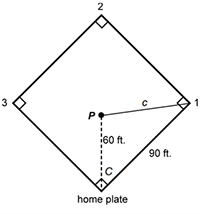The diamond in the shape of a square is oriented such that its diagonals have vertical and horizontal alignments. The bottom vertex is labeled home plate, and moving counterclockwise, the other vertices are labeled 1, 2 and 3. Right angles are shown at each vertex, and a dash line extends along the diagonal of the square from home plate to point P. That line is labeled 60 feet, and an edge of the square is labeled 90 feet.
A triangle is formed by drawing a line, c, from point P to the vertex of the square labeled 1. The included angle between the 60 ft. and 90 ft. segments is labeled C.

The length of two sides and an included angle of the triangle is known, so the Law of Cosines can be used to find the distance between point P and first base. The included angle is one half of a 90-degree angle, so the measure of the included angle, C, is 45 degrees. The Law of Cosines states: c squared equals a squared plus b squared minus 2 times a times b times the Cosine of C. Using a = 60, b = 90, yields c equals the square root of 60 squared plus 90 squared minus 2 times 60 times 90 times the Cosine of 45 degrees is approximately 63.7.

### Competency 0009Understand exponential and logarithmic functions.

9. A certain car that cost 32,000 dollars when new has a depreciated value of 25,600 dollars after one year. Assuming that the value of this car is modeled by an exponential function, what is its value, to the nearest dollar, when the car is 3 years old?

1. 12,800 dollars
2. 13,107 dollars
3. 16,384 dollars
4. 20,480 dollars
Correct Response: C. 25,600 divided by 32,000 equals 0.80, which means that after the first year the car is worth 80% of its original value. Multiplying each side of that equation by 32,000 produces 25,600 equals 32,000 times 0.80. If the car's value continues to decrease in this way, then the value of the car for any year is equal to the initial value (32,000) times a factor of 0.8 for each year since it was purchased. The value of the car, y, after a length of time in years since it was purchased, x, can be determined using the expression y equals 32,000 times 0.8 to the power of x. For x = 3, 32,000 times 0.8 to the power of 3 is approximately 16,384.

### Competency 0010Understand rational, radical, absolute value, and piecewise defined functions.

10. Which of the following statements best describes the graph of the function f of x is a rational function with a numerator of 2 x squared minus 2 and a denominator of x squared plus 3 x plus 2

1. The graph has two x-intercepts, no horizontal asymptote, and one vertical asymptote.
2. The graph has two x-intercepts, horizontal asymptote y = 1, and two vertical asymptotes.
3. The graph has one x-intercept, horizontal asymptote y = 2, and two vertical asymptotes.
4. The graph has one x-intercept, horizontal asymptote y = 2, one vertical asymptote, and one removable discontinuity.
Correct Response: D. The function can be factored to produce f of x equals a rational function with a numerator of 2 times quantity x squared minus 1 and a denominator of quantity x plus 1 times quantity x plus 2. The numerator can be written as 2 times quantity x minus 1 times quantity x plus 1 and a denominator of quantity x plus 1 times quantity x plus 2. The function simplifies to numerator 2 times quantity x minus 1 and denominator x plus 2. Solving the equation 0 equals numerator 2 times quantity x minus 1 and denominator x plus 2 for x leads to one x intercept at x = 1. The value of the fraction numerator x minus 1 and denominator x plus 2 approaches, but never equals, 1 for large values of x. Similarly, the product 2 times numerator x minus 1 and denominator x plus 2 approaches, but never equals, 2, which leads to a horizontal asymptote at y = 2. Since the function is undefined for x equals negative 2, there is a removable discontinuity at x equals negative 2.

### Competency 0011Understand principles and techniques of statistics.

11. Use the histogram below to answer the question that follows.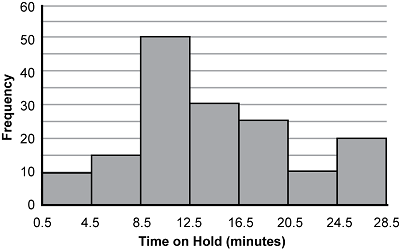The interval from 0.5 to 4.5 minutes has a frequency of 10, 4.5 to 8.5 is 15, 8.5 to 12.5 is 50, 12.5 to 16.5 is 30, 16.5 to 20.5 is 25, 20.5 to 24.5 is 10, and 24.5 to 28.5 is 20.

The automated phone system of a social service agency puts callers on hold to wait for the next representative. Data collected regarding the length of time a caller is on hold are rounded to the nearest minute and are summarized in the histogram. Which of the following conclusions about these data is true?

1. More than half of the callers were on hold for 9 to 12 minutes.
2. Ten percent of the callers were on hold for 21 to 24 minutes.
3. The median time a caller was on hold is in the 13- to 16-minute range.
4. There is a greater chance of being on hold for 25 minutes or more than there is of being on hold for 8 minutes or less.
Correct Response: C. The median is the middle value in an ordered data set. To find the median in a histogram, we can analyze the frequency of each interval as a list: 10, 15, 50, 30, 25, 10, 20. Data values can be removed from both ends of the list simultaneously to identify the median. For instance, simultaneously removing 30 values from each end of the data set changes the list to 0, 0, 45, 30, 25, 0, 0. Removing another 25 values from each end changes the list to 0, 0, 20, 30, 0, 0, 0. Removing another 20 from each end of the data set produces the list 0, 0, 0, 10, 0, 0, 0. At this point, it can be concluded that the median exists within the interval of 13–16 minutes.

### Competency 0012Understand principles and techniques of probability.

12. A container contains two red marbles and three blue marbles. Two students play a game in which they take turns randomly drawing a marble from the container without replacement. The first student to draw a red marble wins the game. What is the probability that the student who goes first will be the winner?

1. one fifth
2. two fifths
3. three fifths
4. four fifths